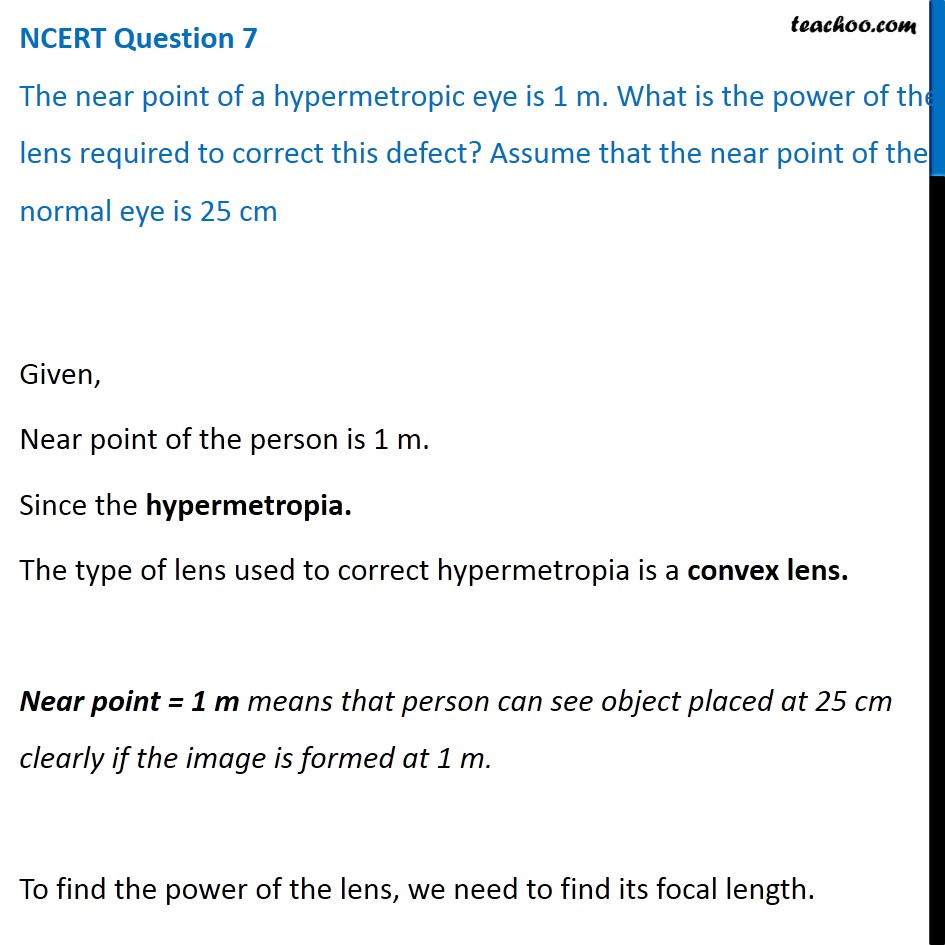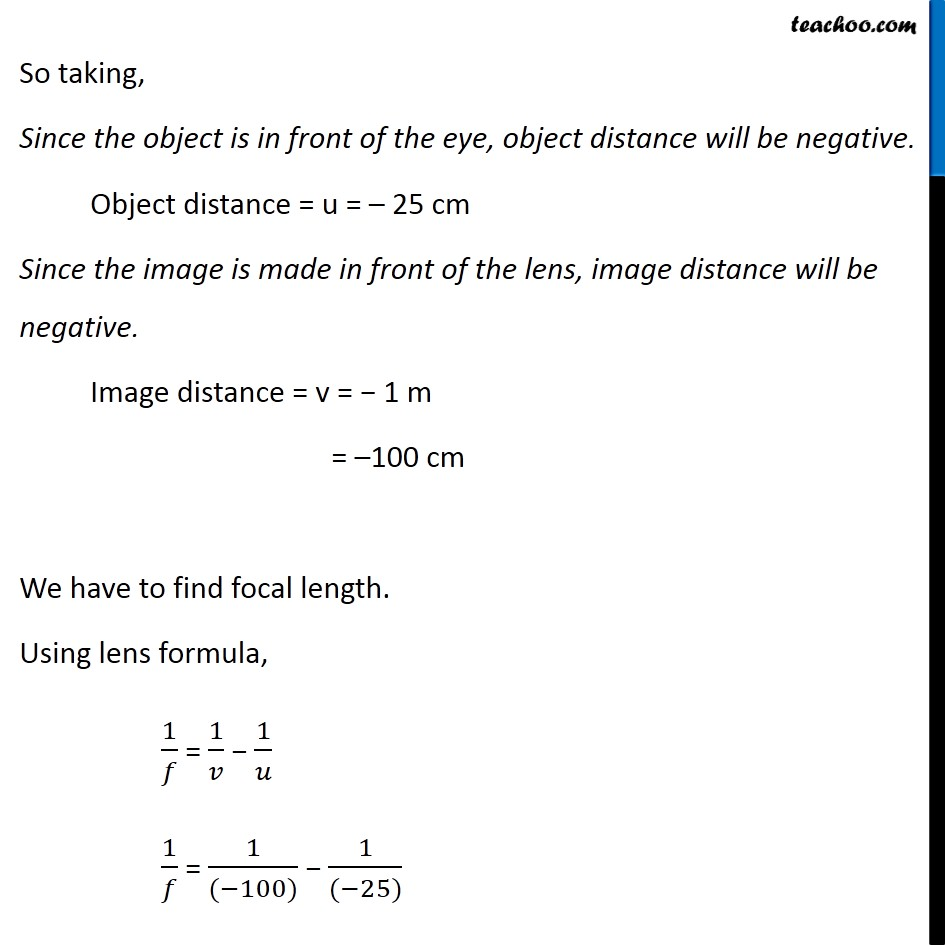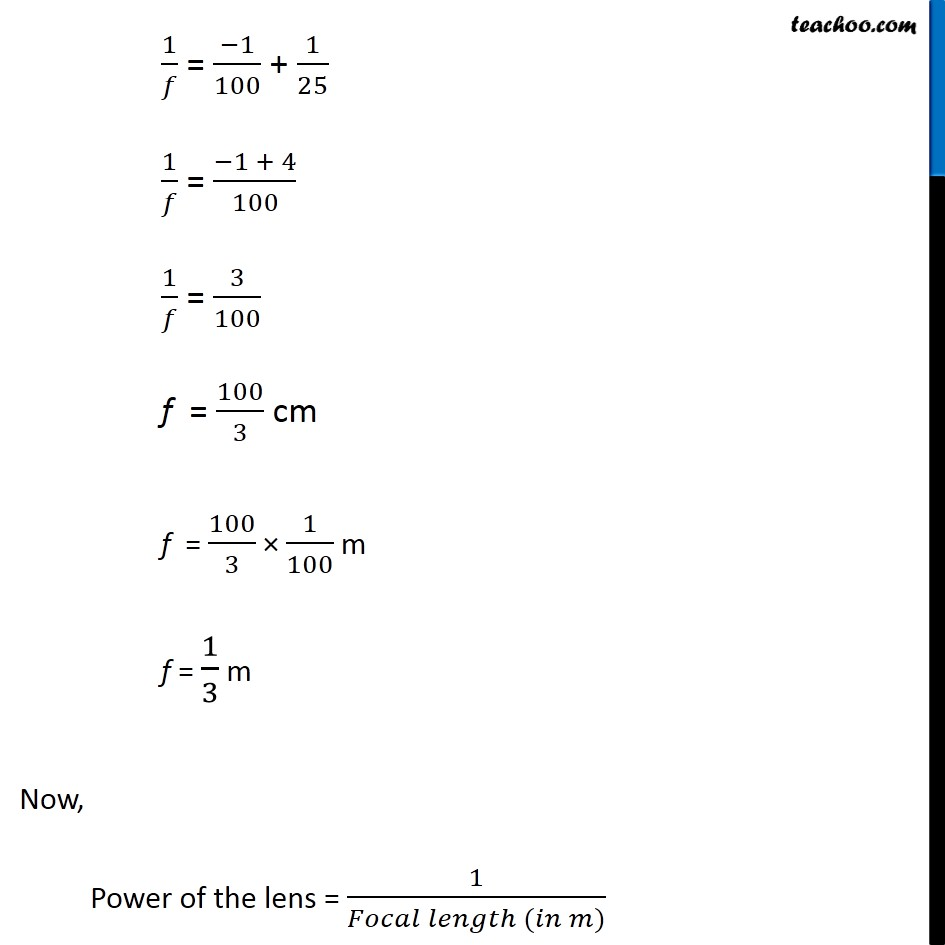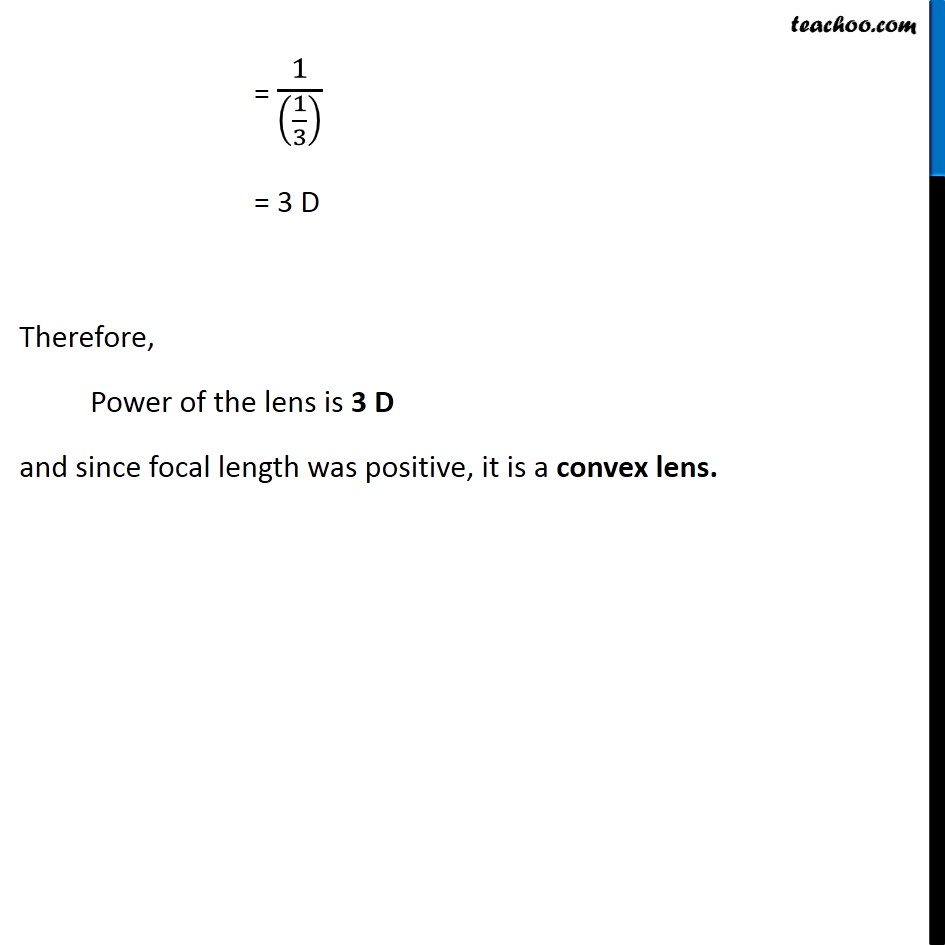NCERT Questions

Class 10
Chapter 11 Class 10 - Human Eye and Colourful World

## Make a diagram to show how hypermetropia is corrected. The near point of a hypermetropic eye is 1 m. What is the power of the lens required to correct this defect? Assume that the near point of the normal eye is 25 cm.Get live Maths 1-on-1 Classs - Class 6 to 12

### Transcript

NCERT Question 7 The near point of a hypermetropic eye is 1 m. What is the power of the lens required to correct this defect? Assume that the near point of the normal eye is 25 cm Given, Near point of the person is 1 m. Since the hypermetropia. The type of lens used to correct hypermetropia is a convex lens. Near point = 1 m means that person can see object placed at 25 cm clearly if the image is formed at 1 m. To find the power of the lens, we need to find its focal length. So taking, Since the object is in front of the eye, object distance will be negative. Object distance = u = – 25 cm Since the image is made in front of the lens, image distance will be negative. Image distance = v = − 1 m = –100 cm We have to find focal length. Using lens formula, 1/𝑓 = 1/𝑣 − 1/𝑢 1/𝑓 = 1/((−100)) − 1/((−25)) 1/𝑓 = (−1)/100 + 1/25 1/𝑓 = (−1 + 4)/100 1/𝑓 = 3/100 f = 100/3 cm f = 100/3 × 1/100 m f = 1/3 m Now, Power of the lens = 1/(𝐹𝑜𝑐𝑎𝑙 𝑙𝑒𝑛𝑔𝑡ℎ (𝑖𝑛 𝑚)) = 1/((1/3) ) = 3 D Therefore, Power of the lens is 3 D and since focal length was positive, it is a convex lens.